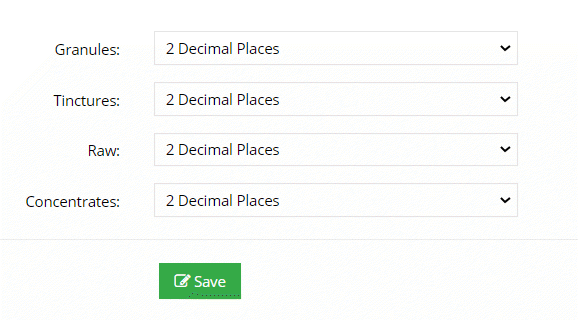# BLOG

Have a Question?

### Decimal Places

Admin > Settings > Formulas > Formula Builder > Decimals

You can specify how many decimal places your formulas will use to calculate total weight received in a formula.  For example, for raw herb formulas, you may want to only allow whole numbers to be added, whereas granules you may want the formula to calculate to the first decimal place.   You can choose per herb type, your maximum number of decimals.